# sklearn.preprocessing.KBinsDiscretizer¶

class sklearn.preprocessing.KBinsDiscretizer(n_bins=5, *, encode='onehot', strategy='quantile', dtype=None, subsample='warn', random_state=None)[source]

Bin continuous data into intervals.

Read more in the User Guide.

New in version 0.20.

Parameters:
n_binsint or array-like of shape (n_features,), default=5

The number of bins to produce. Raises ValueError if n_bins < 2.

encode{‘onehot’, ‘onehot-dense’, ‘ordinal’}, default=’onehot’

Method used to encode the transformed result.

• ‘onehot’: Encode the transformed result with one-hot encoding and return a sparse matrix. Ignored features are always stacked to the right.

• ‘onehot-dense’: Encode the transformed result with one-hot encoding and return a dense array. Ignored features are always stacked to the right.

• ‘ordinal’: Return the bin identifier encoded as an integer value.

strategy{‘uniform’, ‘quantile’, ‘kmeans’}, default=’quantile’

Strategy used to define the widths of the bins.

• ‘uniform’: All bins in each feature have identical widths.

• ‘quantile’: All bins in each feature have the same number of points.

• ‘kmeans’: Values in each bin have the same nearest center of a 1D k-means cluster.

For an example of the different strategies see: Demonstrating the different strategies of KBinsDiscretizer.

dtype{np.float32, np.float64}, default=None

The desired data-type for the output. If None, output dtype is consistent with input dtype. Only np.float32 and np.float64 are supported.

New in version 0.24.

subsampleint or None, default=’warn’

Maximum number of samples, used to fit the model, for computational efficiency. Defaults to 200_000 when strategy='quantile' and to None when strategy='uniform' or strategy='kmeans'. subsample=None means that all the training samples are used when computing the quantiles that determine the binning thresholds. Since quantile computation relies on sorting each column of X and that sorting has an n log(n) time complexity, it is recommended to use subsampling on datasets with a very large number of samples.

Changed in version 1.3: The default value of subsample changed from None to 200_000 when strategy="quantile".

Changed in version 1.5: The default value of subsample changed from None to 200_000 when strategy="uniform" or strategy="kmeans".

random_stateint, RandomState instance or None, default=None

Determines random number generation for subsampling. Pass an int for reproducible results across multiple function calls. See the subsample parameter for more details. See Glossary.

New in version 1.1.

Attributes:
bin_edges_ndarray of ndarray of shape (n_features,)

The edges of each bin. Contain arrays of varying shapes (n_bins_, ) Ignored features will have empty arrays.

n_bins_ndarray of shape (n_features,), dtype=np.int_

Number of bins per feature. Bins whose width are too small (i.e., <= 1e-8) are removed with a warning.

n_features_in_int

Number of features seen during fit.

New in version 0.24.

feature_names_in_ndarray of shape (n_features_in_,)

Names of features seen during fit. Defined only when X has feature names that are all strings.

New in version 1.0.

Binarizer

Class used to bin values as 0 or 1 based on a parameter threshold.

Notes

For a visualization of discretization on different datasets refer to Feature discretization. On the effect of discretization on linear models see: Using KBinsDiscretizer to discretize continuous features.

In bin edges for feature i, the first and last values are used only for inverse_transform. During transform, bin edges are extended to:

np.concatenate([-np.inf, bin_edges_[i][1:-1], np.inf])


You can combine KBinsDiscretizer with ColumnTransformer if you only want to preprocess part of the features.

KBinsDiscretizer might produce constant features (e.g., when encode = 'onehot' and certain bins do not contain any data). These features can be removed with feature selection algorithms (e.g., VarianceThreshold).

Examples

>>> from sklearn.preprocessing import KBinsDiscretizer
>>> X = [[-2, 1, -4,   -1],
...      [-1, 2, -3, -0.5],
...      [ 0, 3, -2,  0.5],
...      [ 1, 4, -1,    2]]
>>> est = KBinsDiscretizer(
...     n_bins=3, encode='ordinal', strategy='uniform', subsample=None
... )
>>> est.fit(X)
KBinsDiscretizer(...)
>>> Xt = est.transform(X)
>>> Xt
array([[ 0., 0., 0., 0.],
[ 1., 1., 1., 0.],
[ 2., 2., 2., 1.],
[ 2., 2., 2., 2.]])


Sometimes it may be useful to convert the data back into the original feature space. The inverse_transform function converts the binned data into the original feature space. Each value will be equal to the mean of the two bin edges.

>>> est.bin_edges_
array([-2., -1.,  0.,  1.])
>>> est.inverse_transform(Xt)
array([[-1.5,  1.5, -3.5, -0.5],
[-0.5,  2.5, -2.5, -0.5],
[ 0.5,  3.5, -1.5,  0.5],
[ 0.5,  3.5, -1.5,  1.5]])


Methods

 fit(X[, y, sample_weight]) Fit the estimator. fit_transform(X[, y]) Fit to data, then transform it. get_feature_names_out([input_features]) Get output feature names. Get metadata routing of this object. get_params([deep]) Get parameters for this estimator. Transform discretized data back to original feature space. set_fit_request(*[, sample_weight]) Request metadata passed to the fit method. set_output(*[, transform]) Set output container. set_params(**params) Set the parameters of this estimator. Discretize the data.
fit(X, y=None, sample_weight=None)[source]

Fit the estimator.

Parameters:
Xarray-like of shape (n_samples, n_features)

Data to be discretized.

yNone

Ignored. This parameter exists only for compatibility with Pipeline.

sample_weightndarray of shape (n_samples,)

Contains weight values to be associated with each sample. Only possible when strategy is set to "quantile".

New in version 1.3.

Returns:
selfobject

Returns the instance itself.

fit_transform(X, y=None, **fit_params)[source]

Fit to data, then transform it.

Fits transformer to X and y with optional parameters fit_params and returns a transformed version of X.

Parameters:
Xarray-like of shape (n_samples, n_features)

Input samples.

yarray-like of shape (n_samples,) or (n_samples, n_outputs), default=None

Target values (None for unsupervised transformations).

**fit_paramsdict

Returns:
X_newndarray array of shape (n_samples, n_features_new)

Transformed array.

get_feature_names_out(input_features=None)[source]

Get output feature names.

Parameters:
input_featuresarray-like of str or None, default=None

Input features.

• If input_features is None, then feature_names_in_ is used as feature names in. If feature_names_in_ is not defined, then the following input feature names are generated: ["x0", "x1", ..., "x(n_features_in_ - 1)"].

• If input_features is an array-like, then input_features must match feature_names_in_ if feature_names_in_ is defined.

Returns:
feature_names_outndarray of str objects

Transformed feature names.

Get metadata routing of this object.

Please check User Guide on how the routing mechanism works.

Returns:

A MetadataRequest encapsulating routing information.

get_params(deep=True)[source]

Get parameters for this estimator.

Parameters:
deepbool, default=True

If True, will return the parameters for this estimator and contained subobjects that are estimators.

Returns:
paramsdict

Parameter names mapped to their values.

inverse_transform(Xt)[source]

Transform discretized data back to original feature space.

Note that this function does not regenerate the original data due to discretization rounding.

Parameters:
Xtarray-like of shape (n_samples, n_features)

Transformed data in the binned space.

Returns:
Xinvndarray, dtype={np.float32, np.float64}

Data in the original feature space.

set_fit_request(*, sample_weight: Union[bool, None, str] = '$UNCHANGED$') [source]

Request metadata passed to the fit method.

Note that this method is only relevant if enable_metadata_routing=True (see sklearn.set_config). Please see User Guide on how the routing mechanism works.

The options for each parameter are:

• True: metadata is requested, and passed to fit if provided. The request is ignored if metadata is not provided.

• False: metadata is not requested and the meta-estimator will not pass it to fit.

• None: metadata is not requested, and the meta-estimator will raise an error if the user provides it.

• str: metadata should be passed to the meta-estimator with this given alias instead of the original name.

The default (sklearn.utils.metadata_routing.UNCHANGED) retains the existing request. This allows you to change the request for some parameters and not others.

New in version 1.3.

Note

This method is only relevant if this estimator is used as a sub-estimator of a meta-estimator, e.g. used inside a Pipeline. Otherwise it has no effect.

Parameters:
sample_weightstr, True, False, or None, default=sklearn.utils.metadata_routing.UNCHANGED

Metadata routing for sample_weight parameter in fit.

Returns:
selfobject

The updated object.

set_output(*, transform=None)[source]

Set output container.

See Introducing the set_output API for an example on how to use the API.

Parameters:
transform{“default”, “pandas”}, default=None

Configure output of transform and fit_transform.

• "default": Default output format of a transformer

• "pandas": DataFrame output

• None: Transform configuration is unchanged

Returns:
selfestimator instance

Estimator instance.

set_params(**params)[source]

Set the parameters of this estimator.

The method works on simple estimators as well as on nested objects (such as Pipeline). The latter have parameters of the form <component>__<parameter> so that it’s possible to update each component of a nested object.

Parameters:
**paramsdict

Estimator parameters.

Returns:
selfestimator instance

Estimator instance.

transform(X)[source]

Discretize the data.

Parameters:
Xarray-like of shape (n_samples, n_features)

Data to be discretized.

Returns:
Xt{ndarray, sparse matrix}, dtype={np.float32, np.float64}

Data in the binned space. Will be a sparse matrix if self.encode='onehot' and ndarray otherwise.

## Examples using sklearn.preprocessing.KBinsDiscretizer¶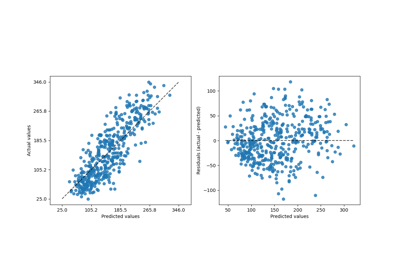Release Highlights for scikit-learn 1.2

Release Highlights for scikit-learn 1.2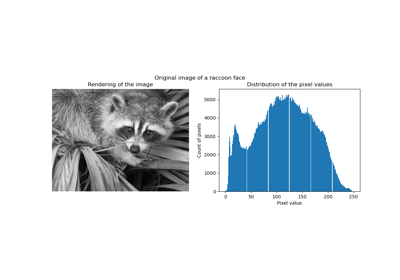Vector Quantization Example

Vector Quantization Example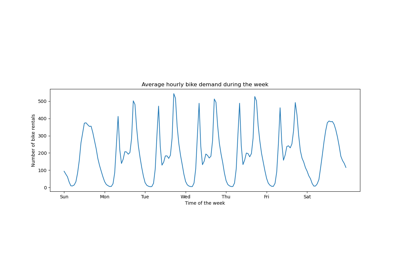Time-related feature engineering

Time-related feature engineering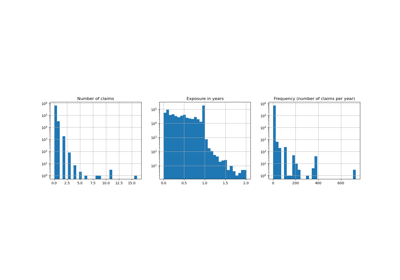Poisson regression and non-normal loss

Poisson regression and non-normal loss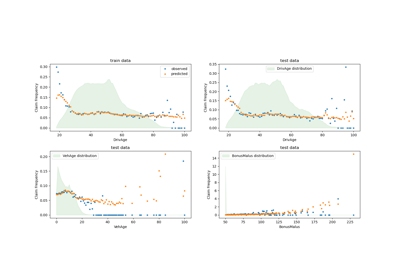Tweedie regression on insurance claims

Tweedie regression on insurance claims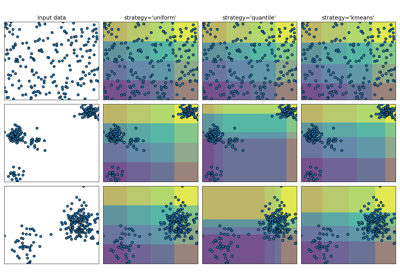Demonstrating the different strategies of KBinsDiscretizer

Demonstrating the different strategies of KBinsDiscretizer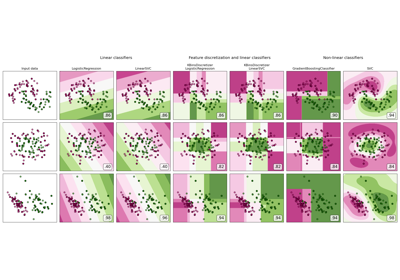Feature discretization

Feature discretization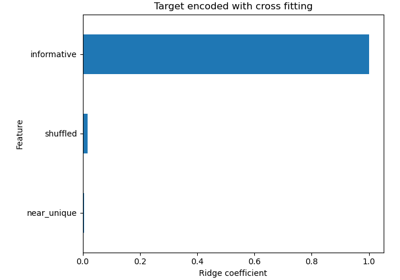Target Encoder’s Internal Cross fitting

Target Encoder's Internal Cross fitting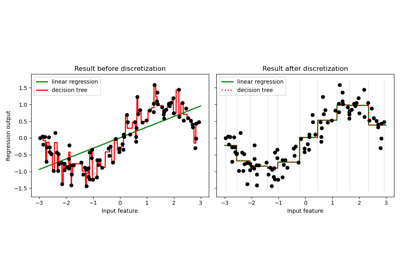Using KBinsDiscretizer to discretize continuous features

Using KBinsDiscretizer to discretize continuous features